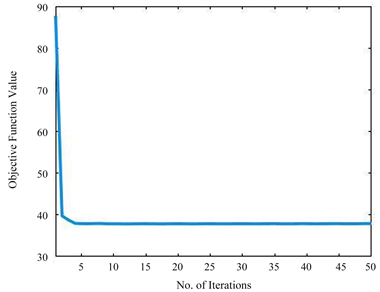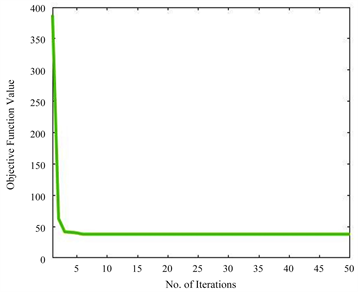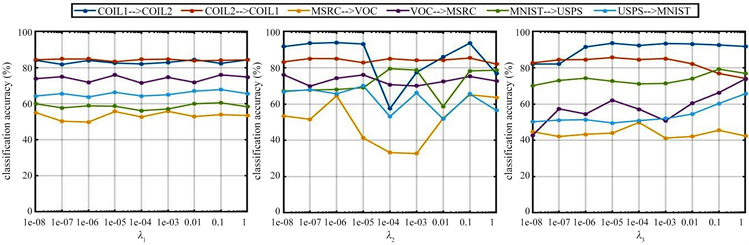﻿ 基于交叉重构的领域自适应算法

Abstract: Domain adaptation is an effective method to solve the problem of cross-domain recognition. It is an effective application of transfer learning in computer vision, which transfers the knowledge learned in the source domain to the recognition task of the target domain, and effectively solves the problem of insufficient labeled data in the target domain. In this paper, a new Cross Reconstruction-based Domain Adaptation (CRDA) method is proposed, which constructs a new source domain and target domain through the cross reconstruction of the original source domain and target domain, so as to make the same kind of data intersect with each other and shorten the distance between the same kind of data. By applying low-rank constraints to the reconstruction matrix, the same kind of data in the two domains are aligned, so as to fully mine the internal structure information between the same kind of data in the source domain and the target domain, and use the structure information to learn the classifier, so as to achieve better cross-domain recognition effect. The experimental results on five open datasets show that CRDA has a high cross-domain recognition accuracy.

1. 引言

2. 相关工作

2.1. 潜在低秩表征

$\begin{array}{c}\underset{Z}{\mathrm{min}}{‖Z‖}_{*}\\ s.t.\text{ }\text{ }\text{ }X=\left[{X}_{O},{X}_{H}\right]Z\end{array}$ (1)

$\begin{array}{c}\underset{Z,E}{\mathrm{min}}{‖Z‖}_{*}+\lambda {‖C‖}_{1}\\ s.t.\text{ }\text{ }\text{ }X=XZ+CX\end{array}$ (2)

$\begin{array}{c}\underset{Z,C,E}{\mathrm{min}}{‖Z‖}_{*}+{‖C‖}_{*}+\lambda {‖E‖}_{1}\\ s.t.\text{ }\text{ }\text{ }X=XZ+CX+E\end{array}$ (3)

2.2. 交叉重构方法

3. 交叉重构的领域自适应方法

3.1. CRDA的目标函数

$\begin{array}{c}\underset{P,Q,Z,E,W}{\mathrm{min}}{‖L-CY‖}_{F}^{2}+{\lambda }_{1}{‖Z‖}_{*}+{\lambda }_{2}{‖E‖}_{2,1}\text{ }+{\lambda }_{3}{‖C‖}_{F}^{2}\\ s.\text{ }t.\text{ }\text{ }\text{ }X=YZ+CY+E\end{array}$ (4)

3.2. CRDA的优化计算方法

$\begin{array}{c}\underset{A,H,Z,C,E}{\mathrm{min}}{‖L-AY‖}_{F}^{2}+{\lambda }_{1}{‖H‖}_{*}+{\lambda }_{2}{‖E‖}_{2,1}\text{ }+{\lambda }_{3}{‖A‖}_{F}^{2}\\ s.\text{ }t.\text{ }\text{ }\text{ }X=YZ+CY+E,\text{ }\text{ }\text{ }Z=H,C=A\end{array}$ (5)

$\begin{array}{c}F\left(A,H,Z,C,E\right)={‖L-AY‖}_{F}^{2}+{\lambda }_{1}{‖H‖}_{*}+{\lambda }_{2}{‖E‖}_{2,1}\text{ }+{\lambda }_{3}{‖A‖}_{F}^{2}+〈{\tau }_{1},X-YZ-CY-E〉\\ \text{\hspace{0.17em}}+〈{\tau }_{2},Z-H〉+〈{\tau }_{3},C-A〉+\frac{\mu }{2}\left({‖X-YZ-CY-E‖}_{F}^{2}+{‖Z-H‖}_{F}^{2}+{‖C-A‖}_{F}^{2}\right)\end{array}$ (6)

$\underset{A}{\mathrm{min}}{‖L-AY‖}_{F}^{2}+{\lambda }_{3}{‖A‖}_{F}^{2}+\frac{\mu }{2}{‖C-A+\frac{{\tau }_{2}}{\mu }‖}_{F}^{2}$ (7)

$F\left(A\right)={‖L-AY‖}_{F}^{2}+{\lambda }_{3}{‖A‖}_{F}^{2}+\frac{\mu }{2}{‖C-A+\frac{{\tau }_{2}}{\mu }‖}_{F}^{2}$，通过令偏导 $\frac{\partial F\left(A\right)}{\partial A}=0$，可以得到A的解如下：

$A=\left[2L{Y}^{\text{T}}+\mu \left(C+\frac{{\tau }_{3}}{\mu }\right)\right]{\left[2Y{Y}^{\text{T}}+\left(2{\lambda }_{3}+\mu \right)E\right]}^{-1}$

$\underset{H}{\mathrm{min}}{\lambda }_{1}{‖H‖}_{*}+\frac{\mu }{2}{‖Z-H+\frac{{\tau }_{2}}{\mu }‖}_{F}^{2}$ (8)

$H={\Theta }_{{\lambda }_{1}/\mu }\left(Z+\frac{{\tau }_{2}}{\mu }\right)$

$\underset{Q}{\mathrm{min}}\frac{\mu }{2}\left({‖X-YZ-CY-E+\frac{{\tau }_{1}}{\mu }‖}_{F}^{2}+{‖Z-H+\frac{{\tau }_{2}}{\mu }‖}_{F}^{2}\right)$ (9)

$F\left(Z\right)=\underset{Q}{\mathrm{min}}\frac{\mu }{2}\left({‖X-YZ-CY-E+\frac{{\tau }_{1}}{\mu }‖}_{F}^{2}+{‖Z-H+\frac{{\tau }_{2}}{\mu }‖}_{F}^{2}\right)$，通过求 $\frac{\partial F\left(Z\right)}{\partial Z}=0$,得到Z的解为：

$Z={\left({Y}^{\text{T}}Y+E\right)}^{-1}\left[{Y}^{\text{T}}\left(X-CY-E+\frac{{\tau }_{1}}{\mu }\right)-\frac{{\tau }_{2}}{\mu }+H\right]$

$\underset{C}{\mathrm{min}}\frac{\mu }{2}\left({‖X-YZ-CY-E+\frac{{\tau }_{1}}{\mu }‖}_{F}^{2}+{‖C-A+\frac{{\tau }_{3}}{\mu }‖}_{F}^{2}\right)$ (10)

$F\left(C\right)=\underset{Q}{\mathrm{min}}\frac{\mu }{2}\left({‖X-YZ-CY-E+\frac{{\tau }_{1}}{\mu }‖}_{F}^{2}+{‖C-A+\frac{{\tau }_{3}}{\mu }‖}_{F}^{2}\right)$，通过求 $\frac{\partial F\left(C\right)}{\partial C}=0$ 得到C的解为：

$C=\left[\left(YZ+E-X-\frac{{\tau }_{1}}{\mu }\right){Y}^{\text{T}}+A-\frac{{\tau }_{3}}{\mu }\right]{\left(I+Y{Y}^{\text{T}}\right)}^{-1}$ (I为单位矩阵)

$\underset{E}{\mathrm{min}}{\lambda }_{2}{‖E‖}_{2,1}+\frac{\mu }{2}{‖X-YZ-CY-E+\frac{{\tau }_{1}}{\mu }‖}_{F}^{2}$ (11)

$\underset{w}{\mathrm{min}}\alpha {‖W‖}_{2,1}+\frac{1}{2}{‖W-Q‖}_{F}^{2}$

${\left[{W}^{*}\right]}_{:,i}\left\{\begin{array}{l}\frac{{‖{Q}_{:,i}‖}_{2}-\alpha }{{‖{Q}_{:,i}‖}_{2}}{Q}_{:,i},\text{ }\text{ }\text{ }\text{ }\text{}当{‖{Q}_{:,i}‖}_{2}>\alpha \text{ }时\\ 0,\text{ }\text{ }\text{ }\text{ }\text{ }\text{ }\text{ }\text{ }\text{ }\text{ }\text{ }\text{ }\text{ }\text{ }\text{ }\text{ }\text{ }\text{ }\text{ }\text{ }\text{ }\text{ }\text{ }\text{ }\text{ }\text{ }\text{ }\text{ }\text{ }\text{ }\text{ }\text{ }\text{ }\text{ }\text{ }\text{ }\text{ }\text{ }\text{ }\text{ }\text{ }\text{ }\text{ }\text{ }\text{ }\text{ }\text{ }\text{ }\text{ }\text{ }\text{ }\text{ }\text{ }\text{ }\text{ }\text{ }\text{ }\text{ }\text{ }\text{ }\text{ }\text{ }\text{ }\text{ }\text{ }\text{ }\text{ }\text{ }\text{ }其他情况\end{array}$

$\frac{{\tau }_{2}}{\mu }$ 替换其中的 $\alpha$，用 $X-YZ-CY+\frac{{\tau }_{1}}{\mu }$ 替换其中的Q，就能够得到E的解

4. 实验

4.1. 数据集介绍

COIL 20数据集：该数据集包含20个不同对象以360度旋转成像。每旋转5度收集一张物体的图像，即每个物体有72幅图像，均为不同角度，共1440张图像。所有的图片裁剪并转换为32 × 32像素的灰度图像。

MSRC & VOC2007数据集：MSRC包含了18个层次的4323幅图像，而VOC 2007包含了20个概念的5011幅图像，它们分别共享了飞机、羊、汽车、牛、鸟、自行车6个语义类，并且将所有图像调整为256像素。

MNIST & USPS数据集：该数据集中有7291幅训练图像和2007幅测试图像；MNIST数据集中有60,000幅训练图像和10,000幅测试图像，这两个数据集共有10个语义类，每个语义类对应数字0~9，所有的图片都被转换为16 × 16像素的灰度图像。

Office & Caltech256数据集：Office数据集是视觉对象识别的基准数据，包括来自三个不同领域的常见对象类别，即A(Amazon)、W(Webcam)和D(DSLR)，每个领域共有31个对象类别。例如笔记本电脑、键盘、显示器、自行车等，一共含有4652张图片。在Amazon域中，每个类别平均有90张图片，而在DSLR或Webcam域中，每个类别平均有30张图片。Caltech-256数据集是用于目标识别的标准数据集，有30,607幅图像和256个类别。

Office-Home数据集：该数据集由来自4个不同领域的图像组成:艺术图像、剪辑艺术、产品图像、现实世界图像，对于每个领域，数据集包含65个对象类别的图像，通常在办公室和家庭中发现。实验中的数据特征是由预先训练好的ResNet50模型提取得到的。

4.2. 超参数设置

4.3. 实验结果和分析

4.3.1. 实验结果Table 1. Experimental results on three different datasetsTable 2. Experimental results on the Office + Caltech datasetTable 3. Experimental results on the Office-Home dataset

4.3.2. 实验结果分析

1). 该方法在大多数数据集上的性能都优于与之比较的方法。这表明，使用改进的类PCA正则化项，CRDA能够很好地保存数据信息。此外，通过对重构矩阵施加低秩约束，可以将来自不同领域但共享同一标签的数据很好的对齐。这就保证了新的特征表示的识别性，因此我们的方法可以显著提高识别准确率。

2). 在高维数据集 Office-home上的实验结果表明，CRDA在大多数高维数据集上表现良好。结果表明，我们的方法能够很好的处理高维度数据。

3). 在半监督领域适应中，人工添加伪标签的对抗式方法是很常见的。在本文中，我们使用原始数据重构代替伪标签来提高分类精度。通过实验，我们发现CRDA的性能比MEDA更好，证明了我们方法的有效性。

4.3.3. 模型收敛性分析(a). COIL20 (COIL2 -> COIL1)(b). Office-Home (Pr -> Ar)

Figure 1. Curve: Model convergence analysis

4.3.4. 参数敏感性分析Figure 2. Curve: The effect of ${\lambda }_{1},{\lambda }_{2},{\lambda }_{3}$ on the classification accuracy

5. 结论

 Weiss, K., Khoshgoftaar, T.M. and Wang, D.D. (2016) A Survey of Transfer Learning. Journal of Big Data, 3, Article No. 9.
https://doi.org/10.1186/s40537-016-0043-6

 Dai, W., Qiang, Y., Xue, G. and Yong, Y. (2007) Boost-ing for Transfer Learning. Proceedings of the 24th International Conference on Machine Learning, Corvallis, 22-24 June 2007, 193-200.
https://doi.org/10.1145/1273496.1273521

 Liu, G. and Yan, S. (2011) Latent Low-Rank Rep-resentation for Subspace Segmentation and Feature Extraction. IEEE International Conference on Computer Vision, Bar-celona, 6-13 November 2011, 1615-1622.
https://doi.org/10.1109/ICCV.2011.6126422

 Pan, Z., Lin, Z. and Chao, Z. (2016) Integrated Low-Rank-Based Discriminative Feature Learning for Recognition. IEEE Transactions on Neural Networks and Learning Systems, 27, 1080-1093.
https://doi.org/10.1109/TNNLS.2015.2436951

 Pan, S.J., Tsang, I.W., Kwok, J.T. and Yang, Q. (2011) Do-main Adaptation via Transfer Component Analysis. IEEE Transactions on Neural Networks, 22, 199-210.
https://doi.org/10.1109/TNN.2010.2091281

 屈磊, 方怡, 熊友玲, 唐俊. 基于L2,1模和图正则化的低秩迁移子空间学习[J]. 控制理论与应用, 2018, 35(12): 1738-1749.

 Georghiades, A.S., Belhumeur, P.N. and Kriegman, D.J. (2002) From Few to Many: Illumination Cone Models for Face Recognition under Variable Lighting and Pose. IEEE Transactions on Pattern Analysis & Machine Intelligence, 23, 643-660.
https://doi.org/10.1109/34.927464

 吴越, 曾向荣, 周典乐, 刘衍, 周家庆, 周经纶. 图像恢复中的稳健交替方向乘子法[J]. 国防科技大学学报, 2018, 40(2): 115-121.

 Yang, J., Yin, W., Zhang, Y. and Wang, Y. (2009) A Fast Algorithm for Edge-Preserving Variational Multichannel Image Restoration. SIAM Journal on Imaging Sciences, 2, 569-592.
https://doi.org/10.1137/080730421

 Gong, B., Yuan, S., Fei, S. and Grauman, K. (2015) Geodesic Flow Kernel for Unsupervised Domain Adaptation. 2012 IEEE Conference on Computer Vision and Pattern Recognition, Providence, 16-21 June 2015, 2066-2073.

 Ming, S., Kit, D. and Yun, F. (2014) Generalized Transfer Subspace Learning through Low-Rank Constraint. International Journal of Computer Vision, 109, 74-93.
https://doi.org/10.1007/s11263-014-0696-6

 Yang, M., Zhang, L., Feng, X. and Zhang, D. (2014) Sparse Representation Based Fisher Discrimination Dictionary Learning for Image Classification. International Journal of Computer Vision, 109, 209-232.
https://doi.org/10.1007/s11263-014-0722-8

 Jing, Z., Li, W. and Ogunbona, P. (2017) Joint Geometrical and Statistical Alignment for Visual Domain Adaptation. CVPR 2017, Honolulu, 21-26 July 2017, 5150-5158.

 Zhu, F. and Shao, L. (2014) Weakly-Supervised Cross-Domain Dictionary Learning for Visual Recognition. International Jour-nal of Computer Vision, 109, 42-59.
https://doi.org/10.1007/s11263-014-0703-y

 Wang, J., Feng, W., Chen, Y., Han, Y. and Yu, P.S. (2018) Visual Domain Adaptation with Manifold Embedded Distribution Alignment. ACM In-ternational Conference on Multimedia, Seoul, 22-26 October 2018, 402-410.
https://doi.org/10.1145/3240508.3240512

 Jia, Z., Ke, G. and Cai, X. (2013) Convergence Analysis of Alter-nating Direction Method of Multipliers for a Class of Separable Convex Programming. Abstract and Applied Analysis, 2013, 205-215.
https://doi.org/10.1155/2013/680768

 Hong, M., Luo, Z.Q. and Razaviyayn, M. (2015) Con-vergence Analysis of Alternating Direction Method of Multipliers for a Family of Nonconvex Problems. SIAM Journal on Optimization, 26, 337-364.
https://doi.org/10.1109/ICASSP.2015.7178689

 Hong, M. and Luo, Z.Q. (2012) On the Linear Convergence of the Alternating Direction Method of Multipliers. Mathematical Programming, 162, 165-199.
https://doi.org/10.1007/s10107-016-1034-2

Top